Derivation of Impedance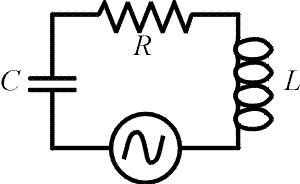We now finish the discussion of the LRC circuit, and derive the expression for the impedance from the previous page. Impedance is the AC analog of resistance. We need to calculate the strength of the current as a function of the voltage of the source. The ratio will yield the impedance. Considering the same circuit on the right, Kirchoff's laws require that the sum of the voltage drops across the three elements equals the voltage of the source at all times. Our goal is to write the sum of these three voltage drops in terms of the current. If the current behaves as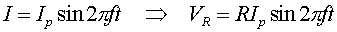the voltage drops across the capacitor and inductor can be written as cosines since displacing a sine function by 90 degrees transforms the function into a cosine. With calculus, one would realize the voltage drops across the inductor and capacitor would have cosine dependence because they are related by derivatives to the current.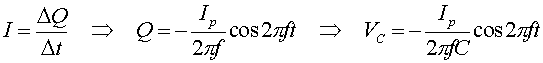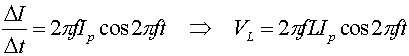We can now sum the voltages to get: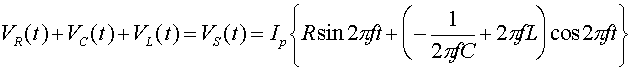Using double-angle formulas, one can rewrite the expression on the right: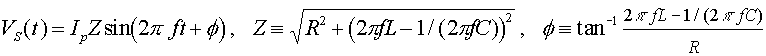The impedance is Z, and the phase difference between the current and the voltage of the source is f.